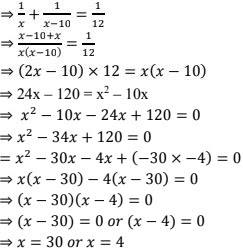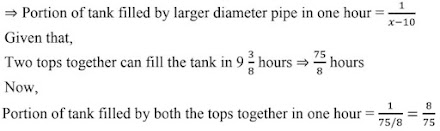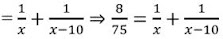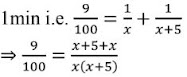# RD Sharma Solutions Chapter 8 Quadratic Equations Exercise 8.12 Class 10 MathsChapter Name RD Sharma Chapter 8 Quadratic Equations Book Name RD Sharma Mathematics for Class 10 Other Exercises Exercise 8.1Exercise 8.2Exercise 8.3Exercise 8.4Exercise 8.5Exercise 8.6Exercise 8.7Exercise 8.8Exercise 8.9Exercise 8.10Exercise 8.11Exercise 8.13 Related Study NCERT Solutions for Class 10 Maths

### Exercise 8.12 Solutions

1. A takes 10 days less than the time taken by B to finish a piece of work. If both A and B together can finish the work in 12 days, find the time taken by B to finish the work.

Solution

Let B takes x days to complete the piece of work.
⇒ B's one days work  = 1/x
Now, A takes 10 days less than that of B to finish the same piece of work i.e.(x - 10) days
⇒ A's one days work = 1/(x - 10)
Given that, both A and B together can finish the same work in 12 days.
⇒ (A and B)'s one days work = 1/12
Now,
(A's one days work) + (B's one days work) = 1/x + 1/(x - 10) and (A + B)'s one days work = 1/12We can observe that, the value of x cannot be less than 10.
∴ The time taken by B to finish the work is 30 days.

2. If two pipes function simultaneously, a reservoir will be filled in 12 hours. One pipe fills the reservoir 10 hours faster than the other. How many hours will the second pipe take to fill the reservoir ?

Solution

Let x be no. of students planned for a picnic
Given that budget for food was Rs 480
⇒ Share of each student = (total budget)/(no. of students) = Rs. 480/x
Given that 8 students foiled to go
⇒ No.of students will be (x - 8)
Now,
Share of each student will be equal to = (total budget )/(No. of students) = Rs. 480/(x -8)
Given that if 8 students failed to go, then cost of food for each member increased by Rs. 10.

3. Two water taps together can fill a tank in 9 hours. The tap of larger diameter takes 10 hours less than the smaller one to fill the tank separately. Find the time in which each tap can separately fill the  tank.

Solution

Let the time taken by the top of smaller diameter to fill the tank be x hours
⇒ Portion of tank filled by smaller pipe in one hour = 1/x
Now, larger diameter pipe takes 10 hours less than that of smaller diameter pipe i.e., (x - 10) hours
⇒ Portion of tank filled by larger diameter pipe in one hour = 1/(x - 10)
Given that,We have,
Portion of tank filled by smaller pipe in 1 hr + Portion of tank filled by larger pipe in 1 hr.4. Two pipes running together can fill a tank in 11 minutes. If one pipe takes 5 minutes more than the other to fill the tank separately, find the time in a which each pipe would fill the tank separately.

Solution

Let us take the time taken by the faster pipe to fill the tank as x minutes.
⇒ Portion of tank filled by faster pipe in one minute = 1/x
Now, time taken by the slower pipe to fill the same tank is 5 minutes more than that of faster pipe i.e.(x + 5) minutes.
⇒ Portion of tank filled by slower pipe in one minute = 1/(x + 5)
Given that
The two pipes together can fill the tank in 11+ 1/9 minutes = 100/9 minutes
⇒ portion of tank filled by faster pipe in 1 min + Portions of tank filled by slower pipe in